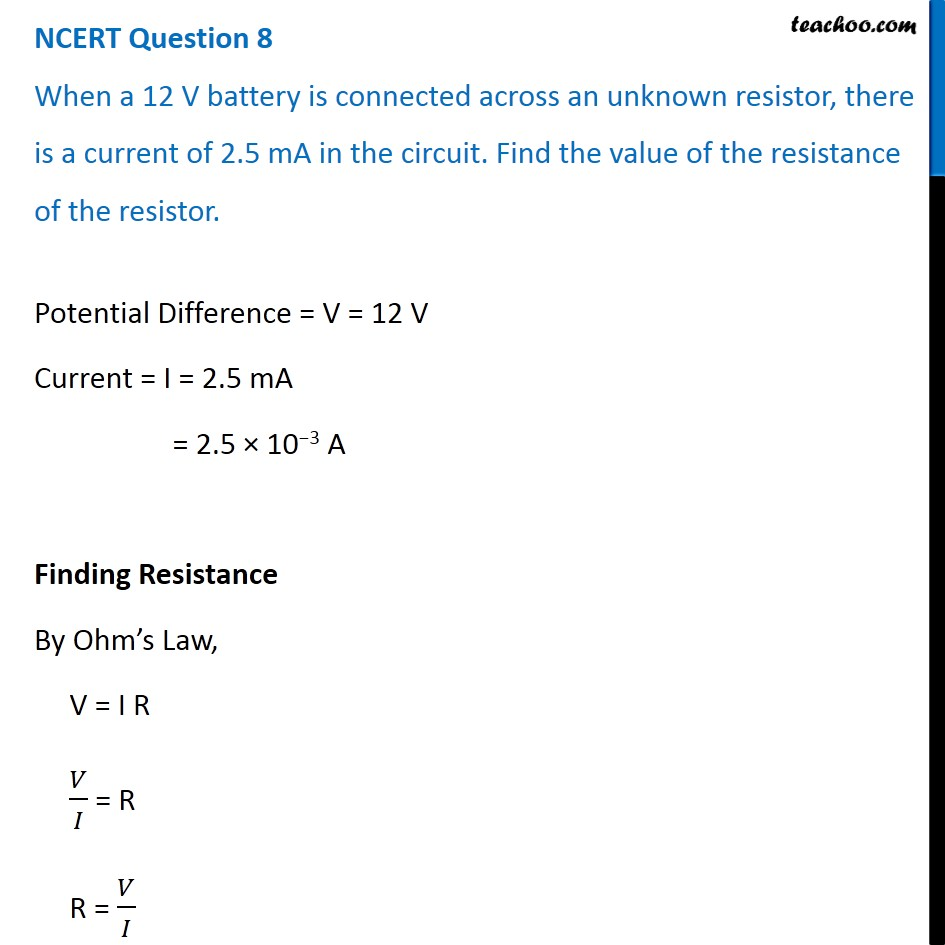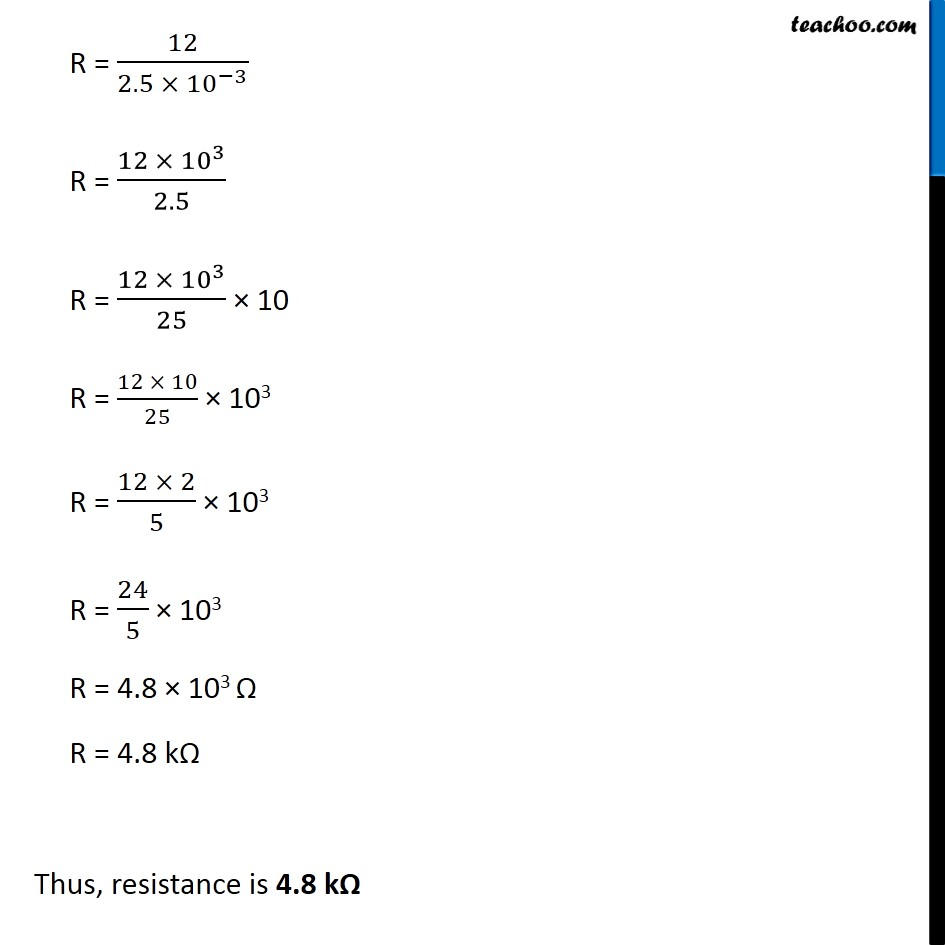NCERT Questions

Class 10
Chapter 12 Class 10 - Electricity

## When a 12 V battery is connected across an unknown resistor, there is a current of 2.5 mA in the circuit. Find the value of the resistance of the resistor.Learn in your speed, with individual attention - Teachoo Maths 1-on-1 Class

### Transcript

NCERT Question 8 When a 12 V battery is connected across an unknown resistor, there is a current of 2.5 mA in the circuit. Find the value of the resistance of the resistor. Potential Difference = V = 12 V Current = I = 2.5 mA = 2.5 × 10−3 A Finding Resistance By Ohm’s Law, V = I R 𝑉/𝐼 = R R = 𝑉/𝐼 R = 12/(2.5 × 〖10〗^(−3) ) R = (12 × 〖10〗^3)/2.5 R = (12 × 〖10〗^3)/25 × 10 R = (12 × 10)/25 × 103 R = (12 × 2)/5 × 103 R = 24/5 × 103 R = 4.8 × 103 Ω R = 4.8 kΩ Thus, resistance is 4.8 kΩ